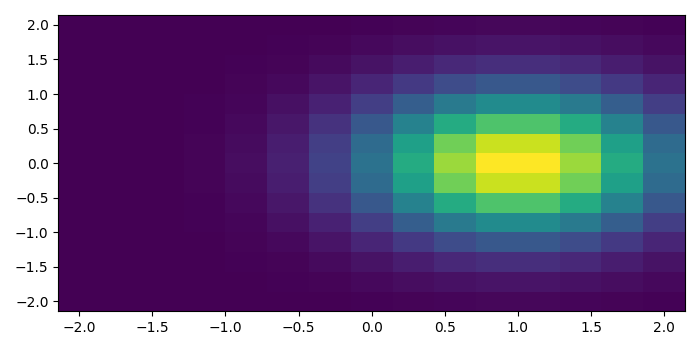# How to plot a density map in Python Matplotlib?

To plot a density map in Python, we can take the following steps −

• Create side, x, y, and z using numpy. Numpy linspace helps to create data between two points based on a third number.

• Return coordinate matrices from coordinate vectors using side data.

• Create exponential data using x and y (Step 2).

• Create a pseudocolor plot with a non-regular rectangular grid using pcolormesh() method.

• To display the figure, use show() method.

## Example

from matplotlib import pyplot as plt, cm, colors
import numpy as np

plt.rcParams["figure.figsize"] = [7.00, 3.50]
plt.rcParams["figure.autolayout"] = True

side = np.linspace(-2, 2, 15)
X, Y = np.meshgrid(side, side)
Z = np.exp(-((X - 1) ** 2 + Y ** 2))

plt.show()